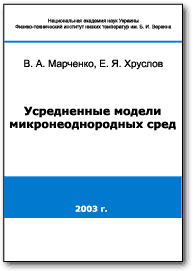Homogenized models of micro-inhomogeneous media Authors: V.A. Marchenko, E.Ya.Khruslov [Main Page] [Summary] [Preface] [Contents]

SummaryProcesses in media, the local properties of which are subject to sharp small scale changes in the space (locally inhomogeneous media) are of great interest in various fields of science and technology, e.g. in the filtration theory, radiophysics, rheology, theory of composite media, etc. Such processes can be described by partial differential equations with rapidly oscillating (in the space variables) coefficients or by boundary value problems in domains with complex microstructure (strongly perforated domains). Because of their complexity these problems cannot be solved by either analytic or numeric methods. But it is remarkable that in many practically important cases, the microscale of the structure is much smaller then the characteristic scale of the process to be studied. Then this process admits a homogenized description in terms of homogenized parameters and equations: coeffcients of these equations are functions smoothly varying in simple domains. These equations can be solved by relatively simple standard methods, numeric or analytic ones, and the coefficients of the homogenized equations are effective rheological parameters of the relative medium.
In the present monograph the asymptotic methods for constructing homogenized models of physical processes in micro-inhomogeneous media (i.e. for obtaining homogenized differential equations and boundary conditions) has been developing. The emphasis is made on the construction of non-standard models for media characterized by several small scale parameters (multiscale models). Rigorous asymptotic analysis (as the small parameters approach zero) shows that such models can be non-local, multicomponent, and even models with memory, depending on the topology of the microstructure. Along with complete proofs of the main results the monograph contains examples of typical structures of micro-inhomogeneous structures and corresponding homogenized models.
The monograph is written primarily for mathematicians: researchers, PhD students and post-doctorants. It will be of use also for physicists, mechanicians, and applied scientists and engineers interesting in problems of rheology and propagation of waves of various nature in micro-inhomogeneous media.